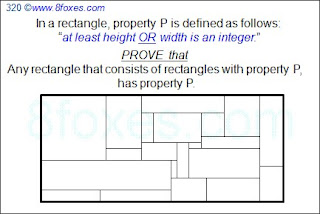## Sunday, January 16, 2011

### Fox 320 - SolutionsThis fox has been discussed extensively. See here...

1. Calculus by Bleaug

He says: "I spotted this problem in a Paul Halmos book "Problems for mathematicians, young and old, 1991", actually in a French translation. He provided the solution below (reformulated by myself) as being proposed by Hugh Montgomery in 1985 in some Math Conference."2. Checkerboard Solution by Rochberg and Stein:

1. Call sub rectangles as TILEs.

2. Start from the lower left corner of the overall rectangle (let's call this point as the origin)Draw horizontal and vertical lines separated by 1/2=0.5 units starting from the origin.

3. This will create 0.5 x 0.5 squares (and possibly rectangles around 2 edges -top and/or RHS)

4. Color the first square (that has the origin as one of its corners) as black the next one as white, and so on to generate a checkerboard-look.

5. Each TILE will have equal areas of black and white (Why?)

6. Therefore the overall rectangle will have equal areas of black and white.

7. So overall rectangle has at least one integer side.

H means the horizontal side is integer, and V means that the vertical side is integer. a1 is the square at the origin. There could have been a smarter combination, but this simple one illustrates the process clearly. This looks like the closest geometric solution we can get -at this time.When there's hardly no day nor hardly no night
There's things half in shadow and halfway in light

3. Induction by Robinson
1. Assume that each H-tile has a width of 1, and each V-tile has a height of 1. Note that any rectangle can be converted this way without distorting the original problem. (This may increase the number of tiles significantly though)

2. Chose any H-tile, say T(0). (If there is no H-tile, then the result is immediate)

3. If there are H-tiles whose lower border shares a segment with T(0)'s upper border, choose one and call it T(1).

4. Otherwise only V-tiles share this border. In this case, we can expand T(0) upward 1 unit. This does not increase the number of H-tiles. Also, the cut V-tiles still have height 1. (They are still V-tiles)

5. Continue expanding T(0) until either the top of the rectangle is reached or a choice of an adjacent H-tile T(1) is possible.

6. Then repeat the same process from T(1). (Continue upward similarly from T(1) to get T(2), and so on...)

7. This will result in a chain of T(0), T(1), T(2), ... , T(m).

8. Starting from T(0) again, work downward similarly to obtain a bigger chain:
T(-n), T(1-n), ... , T(0), T(1), ... , T(m-1), T(m) of H-tiles stretching from bottom to top.

9. Remove these tiles and slide the rest together to get a rectangle with fewer H-tiles.

10. Induction applied to this smaller rectangle yields the result for the original rectangle.

1.This was a great problem, and this proof was nice. I'm sure someone has proved this using the induction method I was trying to do.

2.I'll try to check other proofs from the literature -hopefully- soon.

3.http://www.jstor.org/pss/2322213

Here is a link to the 14 proofs. Unfortunately, you have to pay to see this article.

4.Checkerboard Solution by Rochberg and Stein, was added. Will try to generate a figure later. By the way, yes there are several induction-based solutions too.
-Peace

5.Checkerboard method and bleaug's are two almost-identical solutions. Can anyone else see that?
-Binary

6.damned! you're right. I loved the analytic solution so much that I didn't even try to see what its geometric interpretation was.

Half-unit checkerboard coloring represents a double-periodic function (analog of the exponential in A(R) def). Difference btw black and white areas is the analog of the integral of that function, black being e.g. positive values, white being negative values. A(R)=0 is analog to black and white areas being equal.

Indeed wherever you place the grid over a rectangle with e.g. integer width (grid parallel to sides) it divides the rectangle into back and white strips of equal width (in total). Since these strips have the same total height, the rectangle necessarily has equal black and white areas.

Conversely, we must show that if the grid yields equal black and white areas for some rectangle then either height or width is an integer. Assume rectangle has width=n+x and heigth=m+y, n,m integers, x,y in ]0,1[. Subrectangle [n, m+y] has equal white and black areas so can be ignored. Remaining [x, m+y] rectangle similarly reduces to [x, y] which necessarily will be all black or all white and will be of area x*y>0. Hence such a rectangle cannot have equal black and white areas.

bleaug

7.Yes, Binary's and Bleaug's observations looks correct. The relationship between 2 solutions is like a perfect translation of a statement between two very different languages.

This also implies that if there is another A(R) with the same properties, it can be used to prove the statement. And I think there is.

A picture depicting Checkerboard Solution is added. Enjoy.

-Recpect

8.An induction solution for Tiling a Rectangle by Robinson has just been added.
Six, does this look similar to your approach?

9.Yes, thank you.